## Abstract

The effects of surging and heaving movements of installation platform on mechanical behaviors of SCT installation pipe was studied. Movements of SCT installation platform, ocean wave and current, water depth, SCT weight, installation pipe specification and those dominate the mechanical behaviors of installation pipe and SCT installation precision were considered. The results indicate that surging movement of the platform is the main factor that dominate the deformation of the installation pipe. The surging and heaving movements have significant effect on the tension force loaded on the installation pipe and thus need to be considered when designing SCT installation.

Keywords: SCT installation, installation pipe, mechanical behaviors, movements of platform

## 1. Introduction

Subsea Christmas Trees (SCT) are large structures with facilities to house machinery needed to extract oil and /or natural gas, to inject water or gas into oil reservoir stratum to enrich enhanced oil recovery. However, it has great installation costs and risk. Nowadays, SCT installation using installation pipe through moonpool of the platform are among the most widely used method in the ocean petroleum industry due to its economization and high efficiency. Yet serious risk to operators’ life and economic losses may be caused when a destructive accident happens to the SCT and its installation platform under extreme sea state. The dynamic movements of the SCT and strength of the installation pipe therefore needs to be carefully evaluated in the process of SCT installation design to assure the safety of the structure over the duration of its service life.

The literature offers many research studies on the SCT installation. Almost of them are focused on the installation experiences and technical routes of the SCT installation [1-4]. Other researcher analyzed the mechanical behaviors of the installation pipe under static state. Lin et al. [5-6] established the theoretical model of the SCT installation and analyzed the mechanical behaviors of the installation pipe based on the finite difference method. Wang et al.  analyzed the axial and lateral mechanical behaviors of the SCT installation pipe under static state and the axial dynamic characteristics of the pipe. Hu et al. [8-9] established the theoretical model with time domain of changeable installation pipe and analyzed the lateral movements and strength behaviors of the installation pipe under different installation stages. Qin et al.  established the theoretical analysis model of the installation pipe based on the Euler-Bernoulli beam theory and analyzed the movement and strength behaviors of the pipe considering the variation of wave propagation. Li et al. [11-12] simulated the mechanical behaviors of the installation pipe during SCT passing through the splash zone. Based on the archived documents available to the authors, it seems there are very few research studies investigating the movements of the installation platform on the mechanical behaviors of the SCT installation pipe, although the importance of the dynamic responses of the installation pipe under extreme sea state is recognized by researchers and engineers.

The aim of the research reported here was to investigate the surging and heaving movement of the installation platform, those might affect the strength of installation pipe indicated in our previously researches [13-14], on the dynamic responses of the SCT installation pipe. Movements of SCT installation platform, ocean wave and current, water depth, SCT weight, installation pipe specification and those might dominate the mechanical behaviors of installation pipe and SCT installation precision were considered in this research. It is anticipated that the results of this research may enhance the efforts of researchers and engineers in designing safer and more reliable SCT installation project, therefore, safer and more accurate SCT installation.

## 2. Model development for analysis

### 2.1 Governing equations of SCT installation pipe

The SCT installation pipe is fixed with the installation platform and move along with platform at the top end, shown as in Figure 1. The surging and heaving movement of the platform are considered during the SCT installation process. And the installation pipe is considered as cylinder shell filled with ocean water.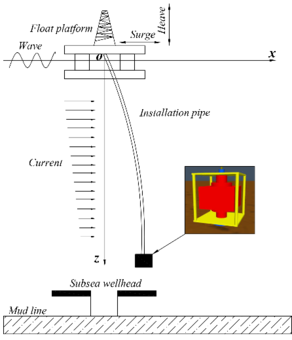Figure 1. Schematic diagram of SCT installation

Shown as in Figure 1, the installation pipe is subjected to wave load, current load conducted by the ocean water and the surging load conducted by the platform at $x$ direction. And the pipe is subjected to the wet weight conducted by SCT and installation pipe and the heaving load conducted by the platform at z direction. Then, the fourth-order partial differential governing equation of the installation can be obtained based on the Euler–Bernoulli beam theory as ,

 $EI{\frac {{\partial }^{4}x}{\partial {z}^{4}}}-T\left(z\right){\frac {{\partial }^{2}x}{\partial {z}^{2}}}+$$\left({m}_{r}+{m}_{w}\right){\frac {{\partial }^{2}x}{\partial {t}^{2}}}+c{\frac {\partial x}{\partial t}}=f\left(z,t\right)$
(1)

where $EI$ is the bending stiffness of the installation pipe, $T(z)$ is the tension force loaded on the installation pipe at water depth of $z$, $m_{r}$ is the mass of each segment of the installation pipe, $m_{w}$ is the mass of fluid inside each segment of pipe, $c$ is the synthetical resistance coefficient and $f(z,t)$ is the environment force loaded on the installation pipe including the wave force, current force and forces conducted by the movement of the installation platform.

The environment loads rarely affect the SCT during the installation process for its small in size and it can be considered as an equivalent particle. Then, the tension force loaded on the installation pipe can be expressed as,

 $T\left(z\right)={G}_{SCR}+\int _{z}^{L}\left(0.25\pi \left({\rho }_{s}-{\rho }_{w}\right)\left({D}_{o}^{2}-{D}_{i}^{2}\right)\right)$
(2)

where $G_{SCT}$ is the wet weight of SCT, $L$ is the length of the installation pipe, $\rho _{s}$ is the density of installation pipe, $\rho _{w}$ is the density of ocean water and $D_{o}$ and $D_{i}$ are the external diameter and internal diameters of the installation pipe. The forces conducted by the wave and current can be obtained based on the Morrison theory as ,

 $f(z,t)=0.5{C}_{D}{\rho }_{w}{D}_{o}\left[{u}_{w}\left(z\right)+{u}_{c}\right]\left|{u}_{w}\left(z\right)+{u}_{c}\right|+0.25\pi {C}_{M}{\rho }_{w}{D}_{o}^{2}{a}_{w}\left(z\right)$
(3)

where $C_{D}$ is the resistance coefficient of ocean water, $u_{w}(z)$ is the horizontal velocity of ocean water at water depth of $z$, $u_{c}$ is the tidal velocity at waterline, $C_{M}$ is the coefficient of inertial force and $a_{w}(z)$ is the horizontal acceleration of ocean water at water depth of $z$. Furthermore, the horizontal velocity and acceleration in the above equation can be obtained as,

 $\left\{{\begin{matrix}{u}_{w}\left({x}_{i}\right)=\displaystyle {\frac {\pi {H}_{w}}{{T}_{w}}}\mathrm {exp} \,\left(-{k}_{n}\cdot {x}_{i}\right)\cdot \mathrm {cos} \,\left({k}_{n}y-{\omega }_{w}t\right)\\{a}_{w}\left({x}_{i}\right)=\displaystyle {\frac {2{\pi }^{2}{H}_{w}}{{T}_{w}^{2}}}\mathrm {exp} \,\left(-{k}_{n}\cdot {x}_{i}\right)\cdot \mathrm {sin} \,\left({k}_{n}y-{\omega }_{w}t\right)\end{matrix}}\right.$
(4)

where $H_{w}$ is wave height of ocean wave, $T_{w}$ is wave period, $k_{n}$ is wave number and $\omega _{w}$ is wave frequency.

### 2.2 Boundary conditions of SCT installation pipe

The SCT installation pipe is fixed with the platform at the top end and move along with the platform. The movement at top end of the installation pipe equal to the movement of the platform. Then, the boundary conditions at top end can be expressed as,

 $\left\{{\begin{matrix}{\left.x\right|}_{z=0}=S(t)\\{\left.{\left.M\right|}_{z=0}=EI\displaystyle {\frac {\partial {x}^{2}}{\partial {z}^{2}}}\right|}_{z=0}={k}_{b}{\left.\displaystyle {\frac {\partial x}{\partial z}}\right|}_{z=0}\end{matrix}}\right.$
(5)

where $S(t)$ is movement of platform along with time, $M$ is the bending moment of installation pipe and $k_{b}$ is connection stiffness. The movement of the platform can be expressed as ,

 $S(t)={S}_{0}+{S}_{L}\mathrm {sin} \,\left({\frac {2\pi t}{{T}_{L}}}-{\alpha }_{L}\right)+\sum _{n=1}^{N}{S}_{n}\mathrm {cos} \,({k}_{n}S(t)-{\omega }_{n}t+{\phi }_{n}+{\alpha }_{n})$
(6)

where ${S}_{0}$ is average offset of platform, ${S}_{L}$ is single amplitude drift of platform, ${T}_{L}$ is drift period of platform, ${\alpha }_{L}$ is the phase angle between ocean wave and platform, ${S}_{n}$ is the single amplitude response of platform, ${\phi }_{n}$ is wave phase angle and ${\alpha }_{n}$ is phase angle between platform and wave period.

The SCT is attached at the bottom end of the installation pipe. The velocity of ocean water is much small at deep water and the effects of ocean wave and current on the installation pipe can be ignored. The inclination slop and shearing force at bottom end equal to zero. Then, the boundary conditions at bottom end can be expressed as,

 $\left\{{\begin{matrix}{\left.{\left.\theta \right|}_{z=L}=\displaystyle {\frac {\partial x}{\partial z}}\right|}_{z=L}=0\\{\left.{\left.Q\right|}_{z=L}=\displaystyle {\frac {{\partial }^{3}x}{\partial {z}^{3}}}\right|}_{z=L}=0\end{matrix}}\right.$
(7)

where $\theta$ is the inclination slop of installation pipe and $Q$ is shearing force loaded on the installation pipe.

## 3. Case study and discussion

### 3.1 Case study

To illustrate the investigated in this research with examples, a platform which is actually in use was selected for analyzing the effects of surging and heaving movements on mechanical behaviors of the SCT installation pipe. Furthermore, the JONSWAP wave was used to simulate the ocean wave loaded on the installation pipe and platform. Figure 2 shows the response amplitude operators (RAO) of the each movement. Table 1 shows the parameters concerned in this case study.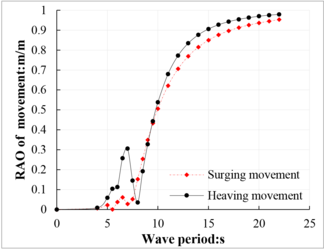Figure 2. RAO of movement of selected platform

Table 1. Parameters of the SCT installation pipe and environment
 Water depth: m 1000 Fluid density inside pipe: kg/m3 1030 External diameter of pipe: m 0.1397 Wet weight of SCT: KN 300 Internal diameter of pipe: m 0.11862 Resistance factor 1.2 Installation pipe density: kg/m3 7850 Inertia coefficient 1 Young's modulus of pipe: GPa 210 Wave height: m 7 Velocity of current at waterline: m/s 1 Wave period: s 8

The displacement of the surging movement and heaving movement under environment are shown in Figures 3 and 4. Apparently, the amplitude of surging displacement of installation platform is much larger than that of amplitude of heaving displacement.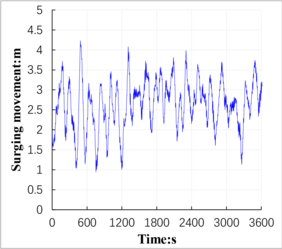Figure 3. Surging displacement of platform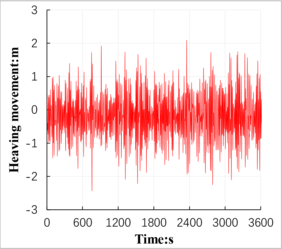Figure 4. Heaving displacement of platform

The SCT installation pipe mainly loaded tension force and bending moment during the SCT installation process. Analysis of surging and heaving movements effects on pipe’s mechanical behaviors should focus on the variation of its deformation, bending stress and effective tension. The performance of the deformation and bending stress are shown as in Figures 5 and 6, respectively. Shown as in Figure 5, the deformation of SCT installation pipe decreases with water depth. The installation pipe deforms between -14.4 m to 15.4 m at top end and deforms between 20.4 m to 21.9 m at bottom end of the pipe. Shown as in Figure 6, the bending stress of the SCT installation pipe decrease with water depth that much consonants with deformation of the pipe. As the maximum stress located at the top end of the installation pipe, the performance of the effective tension force at this node should be carefully analyzed.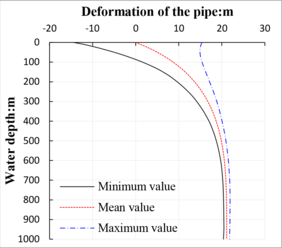Figure 5. Configuration of SCT installation pipe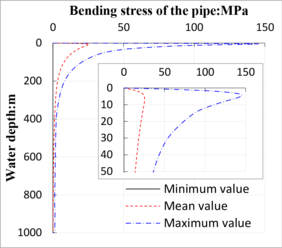Figure 6. Variation of installation pipe’s bending stress

Figure 7 shows the variation of the tension force at top end of the SCT installation pipe along with SCT installation process. The tension force at top of SCT installation pipe varies with SCT installation process. The tension force at top end of the pipe is 587.9 kN at static state (current velocity considered only) obtained in our previously research (RIMNI, 2020; Polish Maritime Research, 2020) while the tension force varies with SCT installation process from 456.2 kN to 726 kN when the surging and heaving movements are considered in this research. Therefore, it is necessary to consider the effects of installation platform’s displacement on installation pipe and the variation of tension force at top end of installation pipe during installing SCT.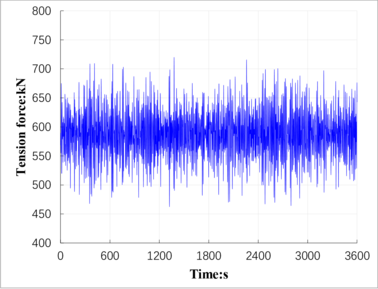Figure 7. Tension force at top end of the installation pipe

The variation of spatial coordinates of SCT need to be accurately determined to assure SCT to be installed correctly above the subsea wellhead on the seabed. The displacement variation of the SCT at $x$ and $z$ direction are shown as in Figures 8 and 9, respectively. Shown as in Figure 8, the displacement of SCT at $x$ direction vary form 20.4m to 21.9m after the surging and heaving movements of platform conducted loads were added on the installation pipe. The displacement of SCT at $z$ direction vary from 994.4 m to 1004.8 m when SCT installation platform’s movements were added on the top end of the installation pipe. Apparently, the variation range of displacement of the SCT at $z$ direction is much larger than that of the variation range at $x$ direction.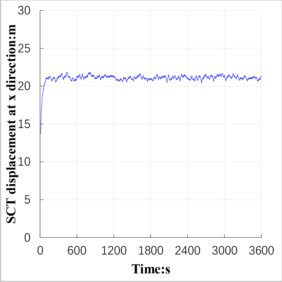Figure 8. Displacement of SCT at $x$ direction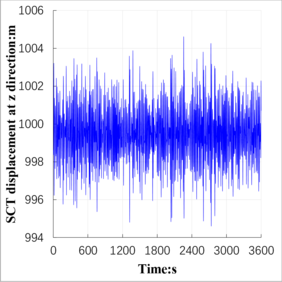Figure 9. Tension force at top end of the installation pipe

## 3.2 Discussion

Effects of water depth. To evaluate the effects of water depth on the mechanical behaviors of SCT installation pipe and SCT displacements, five different water depths were selected ranging from 600m to 1400m in this research. The deformations of the SCT installation pipe in different water depth are shown as in Figure 10(a). Both the minimum and maximum deformations of the installation pipe increase with water depth. As the maximum bending stress of the installation pipe locates at the upper area of the pipe, shown as in Figure 6, this research analyzed the bending stress distribution of areas above the installation pipe. Figure 10(b) shows the bending stress distribution of the installation pipe at upper areas in different water depth. The bending stress of the installation pipe decrease gradually with water depth.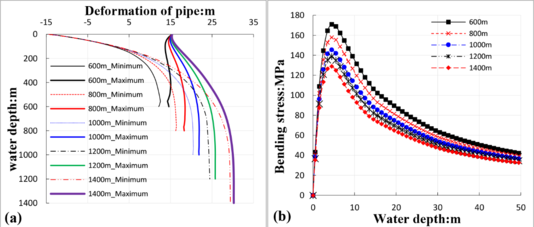Figure 10. Mechanical behaviours of the pipe in different water depths

The displacement of the SCT to be installed above the subsea wellhead on the seabed are shown as in Table 2. Although the displacement of the SCT at $x$ direction increase with water depth, the variation range between maximum value and minimum value decrease with water depth. The displacement of SCT at $z$ direction is smaller than that of the SCT installation platform due to the deformation of the installation pipe at $z$ direction. The tension force loaded at top end of the installation pipe increase with water depth, shown as in Table 3. The maximum tension force loaded on the pipe increased about 23.5% when movements of the platform were considered compared with SCT installed at static state.

Table 2. Displacement of SCT in different water depth
Water Depth: m Displacement at $x$ direction: m Displacement at $z$ direction: m
Minimum Maximum Minimum Maximum
600 12.1 14.6 594.3 604.8
800 16.2 18.3 794.4 804.8
1000 20.4 21.9 994.4 1004.8
1200 24.5 25.8 1194.5 1204.8
1400 29.4 30.25 1394.5 1404.8

Table 3. Tension force loaded at top end of the installation pipe in different water depth
Water depth: m Static state: kN Dynamic state: kN
Minimum Maximum
600 470.4 372.6 573.6
800 529.2 415.4 653.4
1000 587.9 456.2 726.2
1200 646.6 498.6 797.8
1400 705.3 542.2 875.8

Effects of wet weight of SCT. To evaluate the effects of SCT wet weight on the mechanical behaviors of installation pipe and SCT displacements, five different SCT were selected ranging from 200 kN to 400 kN in this research. Figure 11(a) shows the deformations of the SCT installation pipe in different SCT wet weight while Figure 11(b) shows the bending stress distribution of the installation pipe at upper areas in different SCT wet weight. Both deformations and bending stress of the installation pipe decrease with SCT wet weight, which indicate that SCT weight neutralize the deformations and bending stress of the installation pipe.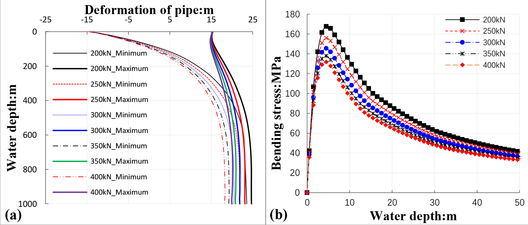Figure 11. Mechanical behaviours of the pipe in different SCT wet weight

Shown as in Table 4, the displacement of SCT at $x$ direction decrease with SCT wet weight while the wet weight of SCT rarely affects the displacement of SCT at $z$ direction. The tension force loaded at top end of the installation pipe increase with SCT wet weight, shown as in Table 5, which indicate that SCT weight needs to be strictly controlled during its design period. The maximum tension force loaded on the pipe increased about 23.4% when movements of the platform were considered compared with SCT installed at static state.

Table 4. Displacement of SCT in different SCT wet weight
SCT wet weight: kN Displacement at $x$ direction: m Displacement at $z$ direction: m
Minimum Maximum Minimum Maximum
200 23.5 24.7 994.3 1004.3
250 21.8 23.1 994.4 1004.6
300 20.4 21.9 994.4 1004.8
350 19.2 20.8 994.5 1005.0
400 18.2 20.0 994.5 1005.0

Table 5. Tension force loaded at top end of the installation pipe in different SCT wet weight
SCT wet weight: kN Static state: kN Dynamic state: kN
Minimum Maximum
200 489.8 377.0 612.0
250 538.8 416.3 670.0
300 587.9 456.2 726.2
350 636.9 494.2 779.5
400 686.0 534.3 834.5

Effects of SCT installation pipe specification. To evaluate the effects of SCT installation pipe specification on the mechanical behaviors of installation pipe and SCT displacements, five different installation pipes (4 in, 4 1/2 in, 5 in, 5 1/2 in and 6 5/8 in) were selected in this research. The displacements of the pipe rarely change with water depth when choosing smaller installation pipe (4 in, 4 1/2 in and 5 in), shown as in Figure 12(a). However, the displacement of SCT installation pipe increases significantly when larger size installation pipe (5 1/2 in and 6 5/8 in) were used in SCT installation process. Similarly, the bending stress of the pipe increase with pipe’s specification when larger installation pipes were selected.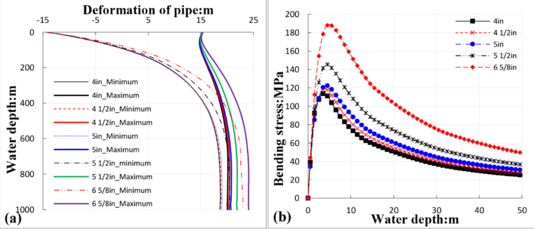Figure 12. Mechanical behaviours of the pipe in different SCT installation pipe

The displacement of SCT at $x$ direction increase with pipe specification while the displacement at $z$ direction decrease slowly with pipe specification, shown as in Table 6. Meanwhile, the tension force loaded on the pipe and variation range of the force increase with pipe’s specification, shown as in Table 7. The maximum tension force loaded on the pipe increased about 22.7% when surging and heaving movements of the platform were considered compared with SCT installed at static state.

Table 6. Displacement of SCT in different installation pipe
Pipe specifications: in Displacement at $x$ direction: m Displacement at $z$ direction: m
Minimum Maximum Minimum Maximum
4 18.6 20.0 994.7 1005.2
4 1/2 18.8 20.4 994.5 1005.0
5 19.0 20.7 994.5 1004.9
5 1/2 20.4 21.9 994.4 1004.8
6 5/8 22.9 24.0 994.4 1004.5

Table 7. Tension force loaded at top end of the installation pipe in different installation pipe
Pipe specifications: in Static state: kN Dynamic state: kN
Minimum Maximum
4 458.3 361.4 557.4
4 1/2 531.33 416.6 645.6
5 599.2 469.3 735.5
5 1/2 587.9 456.2 726.2
6 5/8 601.4 455.6 754.9

## 3. Conclusions

The effects of surging and heaving movements on the mechanical behaviors of SCT installation pipe were investigated in this research. Movements of SCT installation platform, ocean wave and current, water depth, SCT weight, installation pipe specification and those might dominate the mechanical behaviors of installation pipe and SCT installation precision were considered. Based on the research done in this research, the following results and conclusions were obtained:

1. Due to surging movement amplitude is larger than that of the heaving movement of the platform during SCT installation process, the variation range of SCT displacement at $z$ direction is much larger than that of the variation range at $x$ direction.
2. The SCT displacement at $x$ and $z$ direction increase with water depth while its variation range decrease with water depth.
3. The deformation of SCT installation pipe, as well as the bending stress at upper areas of the pipe where locate its maximum stress, increases with water depth and pipe specification while decrease with SCT weight.
4. The maximum tension force loaded at top of the installation pipe increased about 22.7% compared with SCT installed at static state when surging and heaving movements of the platform were considered. And the tension force loaded on the pipe increase with water depth, SCT weight and pipe specification.

## Acknowledgements

The authors acknowledge the support of grant No. 2017QHZ010 from the scientific research-starting project of SWPU China and National Green Remanufacturing System Integration Project Ministry of Industry and Information Technology of China.

### Document informationPublished on 12/01/21
Accepted on 14/12/20
Submitted on 29/10/20

Volume 37, Issue 1, 2021
DOI: 10.23967/j.rimni.2020.12.003

### Document Score0

Views 48
Recommendations 0